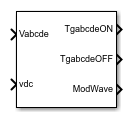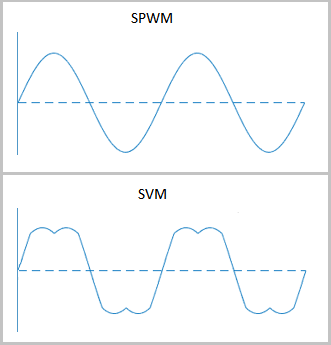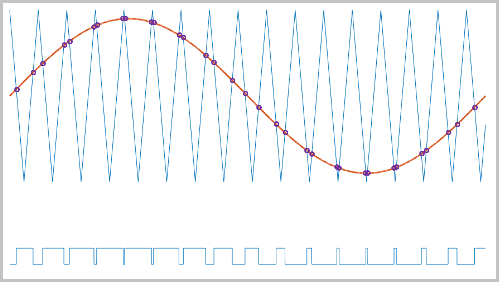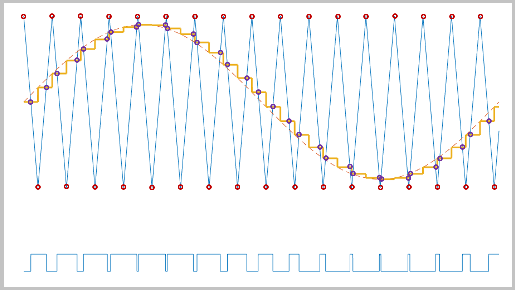# PWM Timing and Waveform Generator (Five-phase, Two-level)

Generate five-phase, two-level gating time information

• Library:
• Simscape / Electrical / Control / Pulse Width Modulation

•## Description

The PWM Timing and Waveform Generator (Five-phase, Two-level) block controls the switching behavior for a five-phase, two-level power converter. The block calculates on-gating and off-gating times and modulation waves based on these block inputs:

• Five sinusoidal reference voltages

This block can be used with average converters to speed up the simulation.

### Continuous PWM

The block only provides continuous pulse width modulation (PWM). The figure shows the general difference between continuous sinusoidal pulse width modulation (SPWM) and continuous space vector modulation (SVM) waveforms.### Sampling Mode

This block allows you to choose natural, symmetric, or asymmetric sampling of the modulation wave.

The PWM Timing and Waveform Generator (Five-phase, Two-level) block does not perform carrier-based PWM. Instead, the block uses input signals to calculate the gating times.

Carrier-based PWM is, however, useful for showing how the sampling mode that you select relates to the switch-on and switch-off behavior of the pulses that the block generates. A generator that uses a two-level, carrier-based PWM method:

1. Samples a reference wave

2. Compares the sample to a triangle carrier wave

3. Generates a switch-on pulse if a sample is higher than the carrier signal or a switch-off pulse if a sample is lower than the carrier wave

To determine switch-on and switch-off pulse behavior, a two-level carrier-based PWM generator uses these methods to sample the triangle wave:

• Natural — The sampling and comparison occur at the intersection points of the modulation wave and the carrier wave.• Asymmetric — Sampling occurs at the upper and lower boundaries of the carrier wave. The comparison occurs at the intersection that follows the sampling.• Symmetric — Sampling occurs at only the upper boundary of the carrier wave. The comparison occurs at the intersection that follows the sampling.## Ports

### Input

expand all

Sinusoidal voltages that you want the attached converter to output, specified as a vector of five elements with one element for each phase.

Positive real number for the DC-link voltage of the converter, specified as a scalar.

### Output

expand all

Switch-on gating time, returned as a vector of five elements with one element for each phase.

Switch-off gating time, returned as a vector of five elements with one element for each phase.

Modulation waves, returned as a vector of five elements, with one element for each phase.

## Parameters

expand all

Continuous pulse width modulation method.

Wave-sampling method. The sampling mode determines whether the block samples the modulation waveform when the waves intersect or when the carrier wave is at one or both of its boundary conditions.

Rate at which the switches in the power converter switch, in Hz.

## Version History

Introduced in R2021a